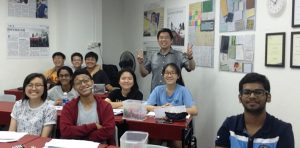# JC H1 & H2 Physics Tuition in SingaporeOur H1 & H2 Physics tuition programme is tailored to help JC students develop a deeper understanding of Physics concepts, master problem-solving techniques, and excel in their academic performance. Our success stems from our tried-and-tested teaching methods, comprehensive resources, and personalised guidance that aims to help each student achieve desired results in the JC Physics exams. As one of the most essential and fundamental fields of science, Physics plays a crucial role in many industries and careers. It is therefore important for students to develop a strong foundation in this subject. Join us on this educational journey, and let us help you achieve your academic goals!

## JC H2 Physics Syllabus (2023)

 1. Measurement 2. Kinematics • Physical quantities and SI units • Scalars and vectors • Errors and uncertainties • Rectilinear motion • Non-linear motion 3. Dynamics 4. Forces • Newton’s laws of motion • Linear momentum and its conservation • Types of force • Centre of gravity • Turning effects of forces • Equilibrium of forces • Upthrust 5. Work, Energy and Power 6. Motion in a Circle • Work • Energy conversion and conservation • Efficiency • Potential energy and kinetic energy • Power • Kinematics of uniform circular motion • Centripetal acceleration • Centripetal force 7. Gravitational Field 8. Temperature and Ideal Gases • Gravitational field • Gravitational force between point masses • Gravitational field of a point mass • Gravitational field near to the surface of the Earth • Gravitational potential • Circular orbits • Thermal equilibrium • Temperature scales • Equation of state • Kinetic theory of gases • Kinetic energy of a molecule 9. First Law of Thermodynamics 10. Oscillations • Specific heat capacity and specific latent heat • Internal energy • First law of thermodynamics • Simple harmonic motion • Energy in simple harmonic motion • Damped and forced oscillations, resonance 11. Wave Motion 12. Superposition • Progressive waves • Transverse and longitudinal waves • Polarisation • Determination of frequency and wavelength of sound waves • Principle of superposition • Stationary waves • Diffraction • Two-source interference • Single slit and multiple slit diffraction 13. Electric Fields 14. Current of Electricity • Concept of an electric field • Electric force between point charges • Electric field of a point charge • Uniform electric fields • Electric potential • Electric current • Potential difference • Resistance and resistivity • Electromotive force 15. D.C. Circuits 16. Electromagnetism • Circuit symbols and diagrams • Series and parallel arrangements • Potential divider • Balanced potentials • Concept of a magnetic field • Magnetic fields due to currents • Force on a current-carrying conductor • Force between current-carrying conductors • Force on a moving charge 17. Electromagnetic Induction 18. Alternating Current • Magnetic flux • Laws of electromagnetic induction • Characteristics of alternating currents • The transformer • Rectification with a diode 19. Quantum Physics 20. Nuclear Physics • Energy of a photon • The photoelectric effect • Wave-particle duality • Energy levels in atoms • Line spectra • X-ray spectra • The uncertainty principle • The nucleus • Isotopes • Nuclear processes • Mass defect and nuclear binding energy • Radioactive decay • Biological effects of radiation

## JC H2 Physics Assessment Exam

PaperTypeDurationScoreWeightage
1Multiple Choice1 Hour3015%
2Structured Questions2 Hours8030%
3Longer Structured Questions2 Hours8035%
4Practical2.5 Hours5520%

## JC H1 Physics Syllabus (2023)

 1. Measurement 2. Kinematics • Physical quantities and SI units • Scalars and vectors • Errors and uncertainties • Rectilinear motion • Non-linear motion 3. Dynamics 4. Forces • Newton’s laws of motion • Linear momentum and its conservation • Types of force • Centre of gravity • Turning effects of forces • Equilibrium of forces 5. Work, Energy and Power 6. Motion in a Circle and Orbits • Work • Energy conversion and conservation • Efficiency • Potential energy and kinetic energy • Power • Kinematics of uniform circular motion • Centripetal acceleration • Centripetal force • Gravitational force between point masses • Circular orbits 7. Current of Electricity 8. D.C. Circuits • Electric current • Potential difference • Resistance and resistivity • Electromotive force • Circuit symbols and diagrams • Series and parallel arrangements • Potential divider 9. Electromagnetism 10. Nuclear Physics • Concept of an electric field • Concept of a magnetic field • Magnetic fields due to currents • Force on a current-carrying conductor • Force between current-carrying conductors • Force on a charge • The nucleus • Isotopes • Nuclear processes • Mass defect and nuclear binding energy • Radioactive decay • Biological effects of radiation

## JC H1 Physics Assessment Exam

PaperTypeDurationScoreWeightage
1Multiple Choice1 Hour3033%
2Structured Questions 2 Hours8067%